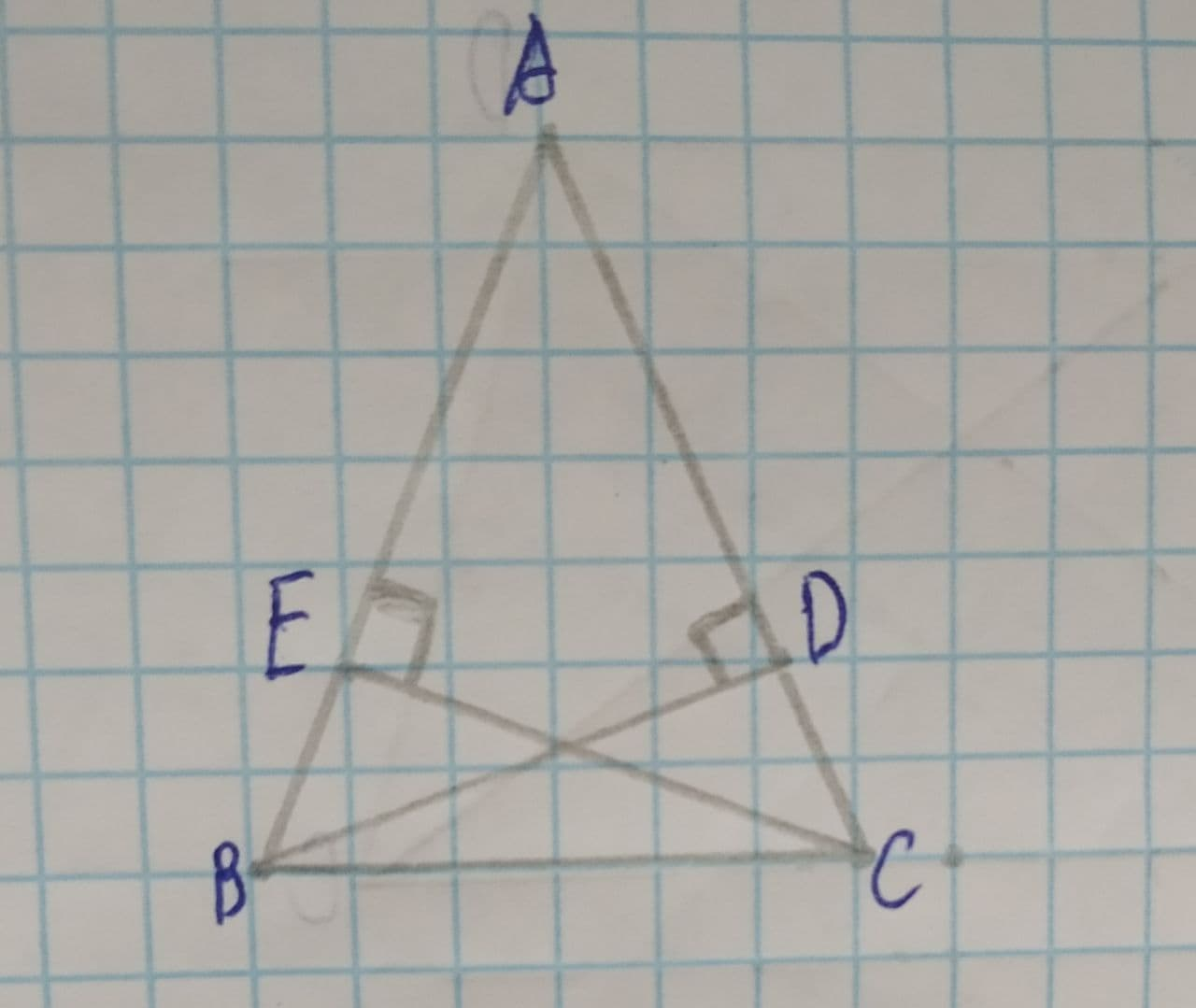Question# \angle ABC\cong \angle ACB, \overline{AE}\perp \overline{EC}\ and\ \overline{AD}\perp \overline{DB} find congruence criteria used to prove that the triangles are congruent.

Congruence
ANSWEREDTo find: congruent criteria used to prove that the triangles are congruent.
Given information:
$$\displaystyle\angle{A}{B}{C}\stackrel{\sim}{=}\angle{A}{C}{B},\overline{{{A}{E}}}\perp\overline{{{E}{C}}}\ {\quad\text{and}\quad}\ \overline{{{A}{D}}}\perp\overline{{{D}{B}}}$$2021-07-26

Consider $$\displaystyle\triangle{B}{A}{D},\triangle{C}{A}{E}$$
As $$\displaystyle\overline{{{A}{D}}}\perp\overline{{{D}{B}}},\angle{B}{D}{A}={90}^{{\circ}}$$
As $$\displaystyle\overline{{{A}{E}}}\perp\overline{{{E}{C}}},\angle{C}{E}{A}={90}^{{\circ}}$$
So,
$$\displaystyle\angle{C}{E}{A}\stackrel{\sim}{=}\angle{B}{D}{A}$$ (i) (right angles are congruent to each other)
Also,
$$\displaystyle\angle{B}{A}{D}\stackrel{\sim}{=}\angle{C}{A}{E}$$ (ii) (common angles in $$\displaystyle\triangle{B}{A}{D},\triangle{C}{A}{E}$$)
As $$\displaystyle\angle{A}{B}{C}\stackrel{\sim}{=}\angle{A}{C}{B}$$,
$$\displaystyle\overline{{{A}{B}}}\stackrel{\sim}{=}\overline{{{A}{C}}}$$ (iii)
(In a triangle, sides opposite to equal angles are equal)
From (i), (ii) and (iii),
$$\displaystyle\triangle{B}{A}{D}\stackrel{\sim}{=}\triangle{C}{A}{E}$$ (by AAS congruence criteria)